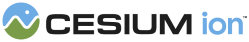#BoundingRectangle

#### new Cesium.BoundingRectangle(x, y, width, height) Core/BoundingRectangle.js 23

A bounding rectangle given by a corner, width and height.
Name Type Default Description
`x` Number `0.0` optional The x coordinate of the rectangle.
`y` Number `0.0` optional The y coordinate of the rectangle.
`width` Number `0.0` optional The width of the rectangle.
`height` Number `0.0` optional The height of the rectangle.

### Members

#### static Cesium.BoundingRectangle.packedLength : Number Core/BoundingRectangle.js 57

The number of elements used to pack the object into an array.

#### height : Number Core/BoundingRectangle.js 50

The height of the rectangle.
Default Value: `0.0`

#### width : Number Core/BoundingRectangle.js 43

The width of the rectangle.
Default Value: `0.0`

#### x : Number Core/BoundingRectangle.js 29

The x coordinate of the rectangle.
Default Value: `0.0`

#### y : Number Core/BoundingRectangle.js 36

The y coordinate of the rectangle.
Default Value: `0.0`

### Methods

#### static Cesium.BoundingRectangle.clone(rectangle, result) → BoundingRectangle Core/BoundingRectangle.js 205

Duplicates a BoundingRectangle instance.
Name Type Description
`rectangle` BoundingRectangle The bounding rectangle to duplicate.
`result` BoundingRectangle optional The object onto which to store the result.
##### Returns:
The modified result parameter or a new BoundingRectangle instance if one was not provided. (Returns undefined if rectangle is undefined)

#### static Cesium.BoundingRectangle.equals(left, right) → Boolean Core/BoundingRectangle.js 331

Compares the provided BoundingRectangles componentwise and returns `true` if they are equal, `false` otherwise.
Name Type Description
`left` BoundingRectangle optional The first BoundingRectangle.
`right` BoundingRectangle optional The second BoundingRectangle.
##### Returns:
`true` if left and right are equal, `false` otherwise.

#### static Cesium.BoundingRectangle.expand(rectangle, point, result) → BoundingRectangle Core/BoundingRectangle.js 264

Computes a bounding rectangle by enlarging the provided rectangle until it contains the provided point.
Name Type Description
`rectangle` BoundingRectangle A rectangle to expand.
`point` Cartesian2 A point to enclose in a bounding rectangle.
`result` BoundingRectangle optional The object onto which to store the result.
##### Returns:
The modified result parameter or a new BoundingRectangle instance if one was not provided.

#### static Cesium.BoundingRectangle.fromPoints(positions, result) → BoundingRectangle Core/BoundingRectangle.js 117

Computes a bounding rectangle enclosing the list of 2D points. The rectangle is oriented with the corner at the bottom left.
Name Type Description
`positions` Array.<Cartesian2> List of points that the bounding rectangle will enclose. Each point must have `x` and `y` properties.
`result` BoundingRectangle optional The object onto which to store the result.
##### Returns:
The modified result parameter or a new BoundingRectangle instance if one was not provided.

#### static Cesium.BoundingRectangle.fromRectangle(rectangle, projection, result) → BoundingRectangle Core/BoundingRectangle.js 167

Computes a bounding rectangle from a rectangle.
Name Type Default Description
`rectangle` Rectangle The valid rectangle used to create a bounding rectangle.
`projection` Object `GeographicProjection` optional The projection used to project the rectangle into 2D.
`result` BoundingRectangle optional The object onto which to store the result.
##### Returns:
The modified result parameter or a new BoundingRectangle instance if one was not provided.

#### static Cesium.BoundingRectangle.intersect(left, right) → Intersect Core/BoundingRectangle.js 299

Determines if two rectangles intersect.
Name Type Description
`left` BoundingRectangle A rectangle to check for intersection.
`right` BoundingRectangle The other rectangle to check for intersection.
##### Returns:
`Intersect.INTERSECTING` if the rectangles intersect, `Intersect.OUTSIDE` otherwise.

#### static Cesium.BoundingRectangle.pack(value, array, startingIndex) → Array.<Number> Core/BoundingRectangle.js 68

Stores the provided instance into the provided array.
Name Type Default Description
`value` BoundingRectangle The value to pack.
`array` Array.<Number> The array to pack into.
`startingIndex` Number `0` optional The index into the array at which to start packing the elements.
##### Returns:
The array that was packed into

#### static Cesium.BoundingRectangle.union(left, right, result) → BoundingRectangle Core/BoundingRectangle.js 234

Computes a bounding rectangle that is the union of the left and right bounding rectangles.
Name Type Description
`left` BoundingRectangle A rectangle to enclose in bounding rectangle.
`right` BoundingRectangle A rectangle to enclose in a bounding rectangle.
`result` BoundingRectangle optional The object onto which to store the result.
##### Returns:
The modified result parameter or a new BoundingRectangle instance if one was not provided.

#### static Cesium.BoundingRectangle.unpack(array, startingIndex, result) → BoundingRectangle Core/BoundingRectangle.js 92

Retrieves an instance from a packed array.
Name Type Default Description
`array` Array.<Number> The packed array.
`startingIndex` Number `0` optional The starting index of the element to be unpacked.
`result` BoundingRectangle optional The object into which to store the result.
##### Returns:
The modified result parameter or a new BoundingRectangle instance if one was not provided.

#### clone(result) → BoundingRectangle Core/BoundingRectangle.js 349

Duplicates this BoundingRectangle instance.
Name Type Description
`result` BoundingRectangle optional The object onto which to store the result.
##### Returns:
The modified result parameter or a new BoundingRectangle instance if one was not provided.

#### equals(right) → Boolean Core/BoundingRectangle.js 370

Compares this BoundingRectangle against the provided BoundingRectangle componentwise and returns `true` if they are equal, `false` otherwise.
Name Type Description
`right` BoundingRectangle optional The right hand side BoundingRectangle.
##### Returns:
`true` if they are equal, `false` otherwise.

#### intersect(right) → Intersect Core/BoundingRectangle.js 359

Determines if this rectangle intersects with another.
Name Type Description
`right` BoundingRectangle A rectangle to check for intersection.
##### Returns:
`Intersect.INTERSECTING` if the rectangles intersect, `Intersect.OUTSIDE` otherwise.
Need help? The fastest way to get answers is from the community and team on the Cesium Forum.